Courses

# NCERT Solutions(Part- 6)- Linear Equations in One Variable Class 8 Notes | EduRev

## Class 8 Mathematics by VP Classes

Created by: Vp Classes

## Class 8 : NCERT Solutions(Part- 6)- Linear Equations in One Variable Class 8 Notes | EduRev

The document NCERT Solutions(Part- 6)- Linear Equations in One Variable Class 8 Notes | EduRev is a part of the Class 8 Course Class 8 Mathematics by VP Classes.
All you need of Class 8 at this link: Class 8

EXERCISE 2.6

Question: Solve the following equations

1.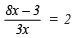2.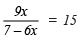3.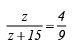4.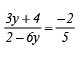5.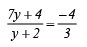Solution:
1.Multiplying both sides by 3x, we have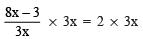or

8x – 3 = 6x

Transposing (–3) to RHS and 6x to LHS, we have

8x – 6x = 3

or

2x = 3

Dividing both sides by 2, we have

x = 3/2

2.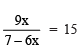Multiplying both sides by 7 – 6x, we have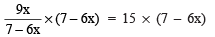or

9x = 105 – 90x

Transposing (–90x) to LHS, we have

9x + 90x = 105

or

99x = 105

or

x = 105/99 (Dividing both sides by 99)

or

x = 35/33

3.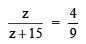By cross multiplication, we have
9z = 4(z + 15) ⇒ 9z = 4z + 60

Transposing 4z to LHS, we have
9z – 4z = 60

5z = 60 ⇒  z = 60/5 = 12

∴   z = 12

4.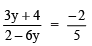By cross multiplication, we have
5(3y + 4) = –2(2 – 6y)

or

15y + 20 = –4 + 12y

Transposing 20 to RHS and 12y to LHS, we have

15y – 12y = –4 – 20

or

3y = –24

or

y = - 24/3= –8    (Dividing both sides by 3)

or   y = –8

5.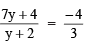By cross multiplication, we have

3 * [7y + 4] = –4 x [y + 2]

or

21y + 12 = –4y – 8

Transposing 12 to RHS and (–4y) to LHS, we have

21y + 4y = –8 – 12

or

25y = –20

or

y = -20/25 (Dividing both sides by 25)

or

y = -20/25 = -4

∴   y = -4/5

Question 6. The ages of Hari and Harry are in the ratio 5 : 7. Four years from now the ratio of their ages will be 3 : 4. Find their present ages.
Solution: Let the present age of Hari = 5x years
and the present age of Harry = 7x years
After 4 years, Age of Hari = (5x + 4) years
Age of Harry = (7x + 4) years

According to the condition,

(5x + 4) : (7x + 4) = 3 : 4

or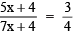By cross multiplication, we have:

4(5x + 4) = 3(7x + 4)

or

20x + 16 = 21x + 12

Transposing 16 to RHS and 21x to LHS, we have

20x – 21x = 12 – 16

–x = –4 ⇒ x = 4

∴ Present age of Hari = 5 * 4 = 20 years

Present age of Harry = 7 * 4 = 28 years

Question 7. The denominator of a rational number is greater than its numerator by 8. If the numerator is increased by 17 and the denominator is decreased by 1, the number obtained is 3/2. Findthe rational number.
Solution: Let the numerator = x

∴ Denominator = x + 8

New numerator = (x) + 17
New denominator = (x + 8) – 1 = x + 7

∴ The new number =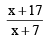According to the condition, we have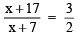By cross multiplication, we have
2(x + 17) = 3(x + 7) 2x + 3x = 3x + 21

Transposing 34 to RHS and 3x to LHS, we have

2x – 34 = 21 – 34 ⇒ –x = –13

∴ x= 13 ⇒ Numerator = 13

x + 8 = 13 + 8 = 21 ⇒Denominator = 21

∴ The rational number = 13/21

Offer running on EduRev: Apply code STAYHOME200 to get INR 200 off on our premium plan EduRev Infinity!

93 docs|16 tests

,

,

,

,

,

,

,

,

,

,

,

,

,

,

,

,

,

,

,

,

,

;